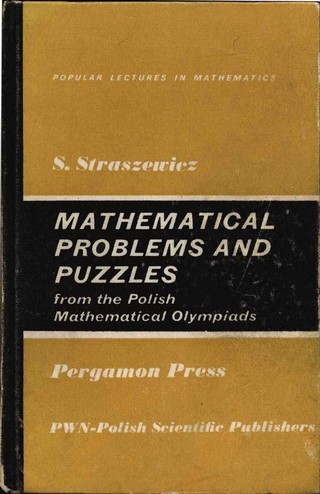### Book Toankho

Sách hay - Sách đẹp

# Mathematical Problems and Puzzles (From the Polish Mathematical Olympiads) (Popular Lectures in Mathematics Vol 12)Popular Lectures in Mathematics, Volume 12: Mathematical Problems and Puzzles: From the Polish Mathematical Olympiads contains sample problems from various fields of mathematics, including arithmetic, algebra, geometry, and trigonometry. The contest for secondary school pupils known as the Mathematical Olympiad has been held in Poland every year since 1949/50. This book is composed of two main parts. Part I considers the problems and solutions about integers, polynomials, algebraic fractions and irrational experience. Part II focuses on the problems of geometry and trigonometric transformation, along with their solutions. The provided solutions aim to extend the student’s knowledge of mathematics and train them in mathematical thinking.
This book will prove useful to secondary school mathematics teachers and students.
Download ToanKho:
http://lk.libvui.com/mwNma
http://megaurl.io/fdPzr

loading...
Loading...